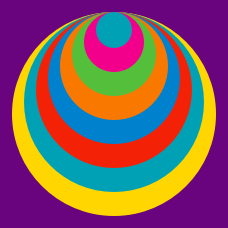Geometry

# Composite Figures: Level 2 Challenges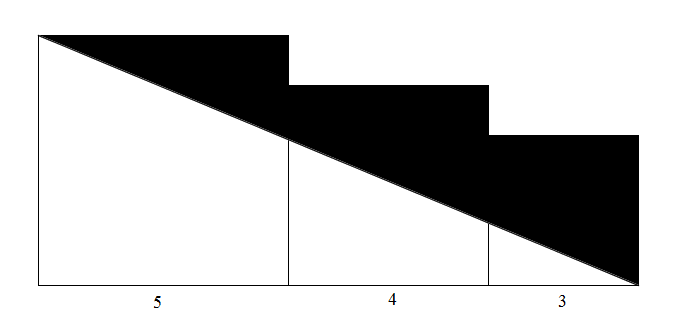The figure above shows three consecutive squares of sides 5, 4 and 3, respectively.

Find the area of the black region.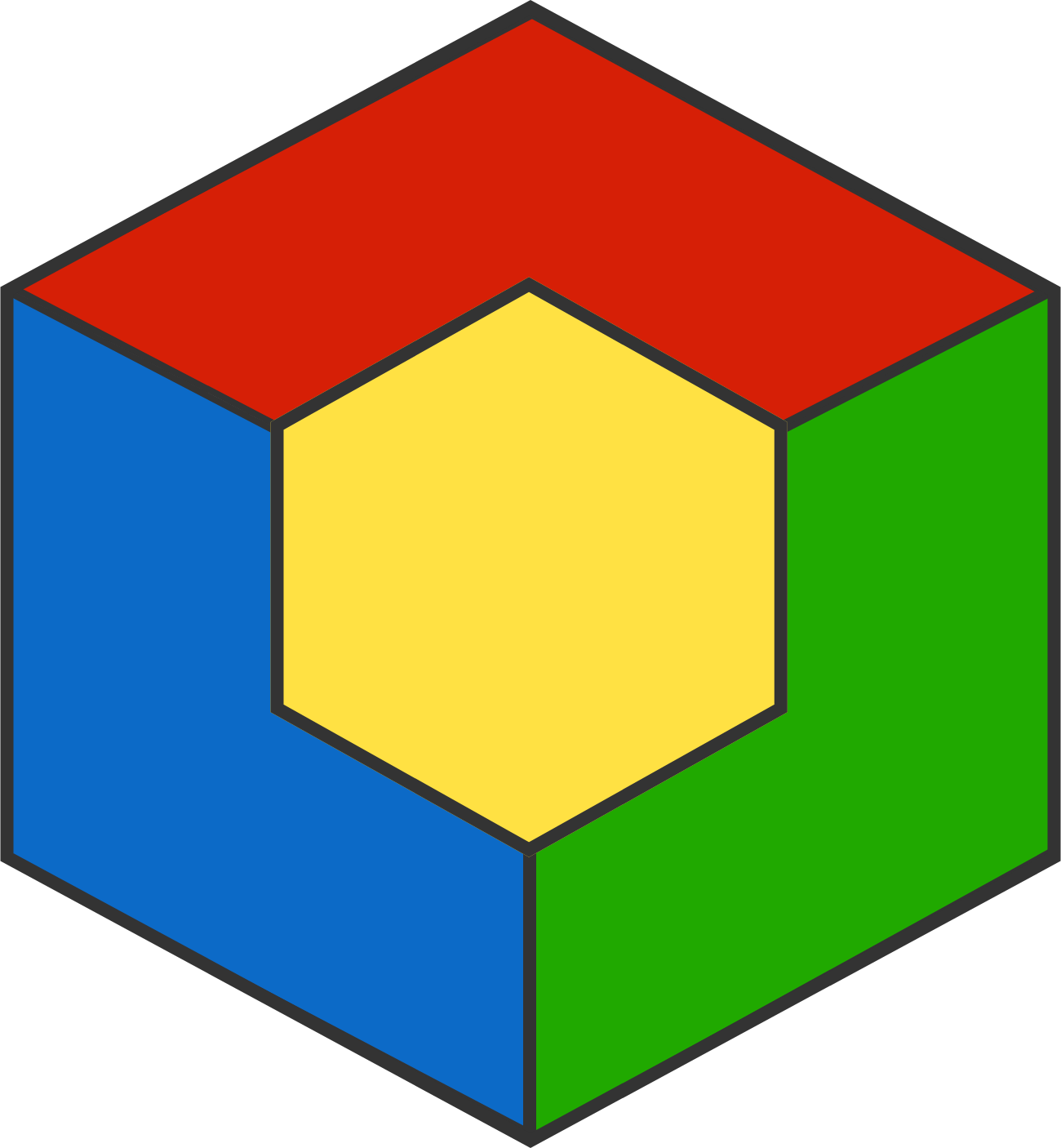In the diagram above, a yellow regular hexagon lies inside a larger regular hexagon with twice as large a side length.

Each of the 3 line segments connecting the inner and outer hexagons has the same length as a side of the yellow hexagon.

Is it true that the 4 colored regions have the same area?

The line segments shown divide parallelogram $ABCD$ into 8 regions, and the areas of the orange triangles are 48, 21, and 63.

What is the area of the blue triangle?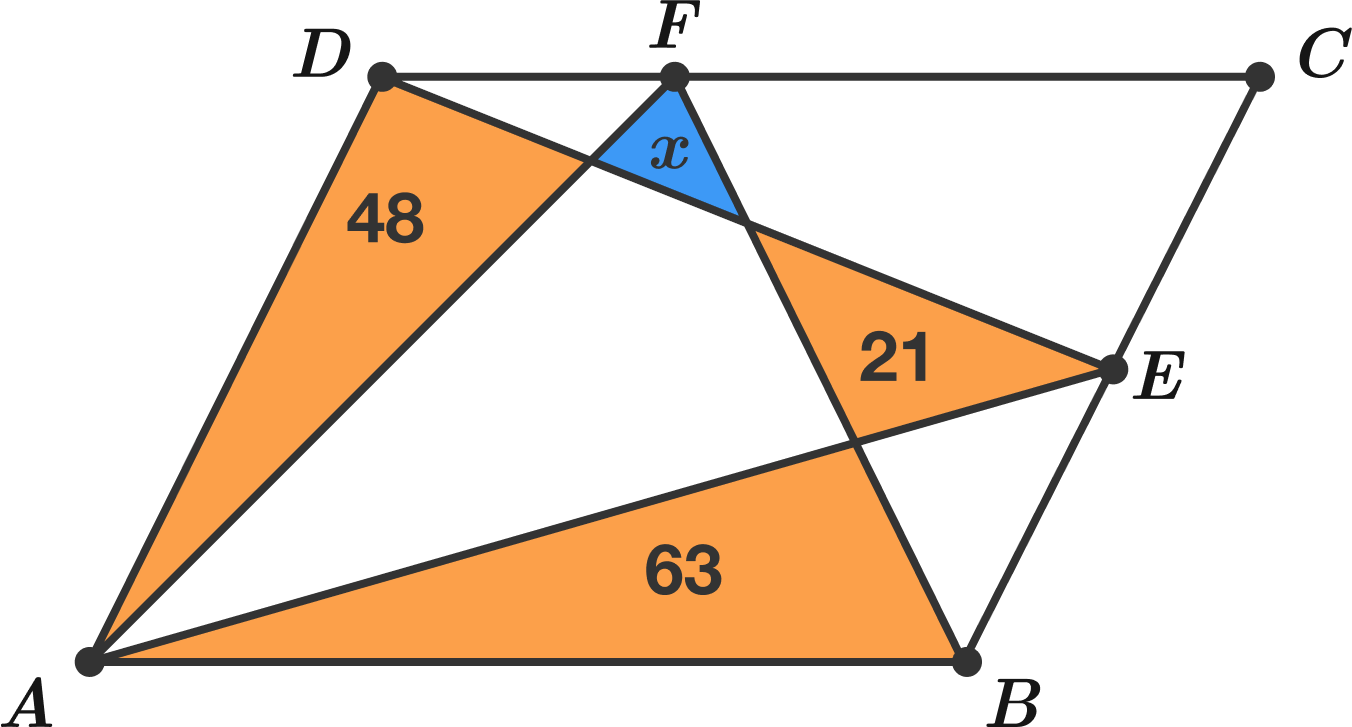Draw a $1\text{ m}$ by $1\text{ m}$ square.
Then inscribe the largest possible circle within the square previously drawn.
Then inscribe the largest possible square within the circle previously drawn.
Then inscribe the largest possible circle within the square previously drawn.
Then inscribe the largest possible square within the circle previously drawn.
Then repeat the process indefinitely.

What will be the total area of all the squares drawn (in $\text{m}^2$)?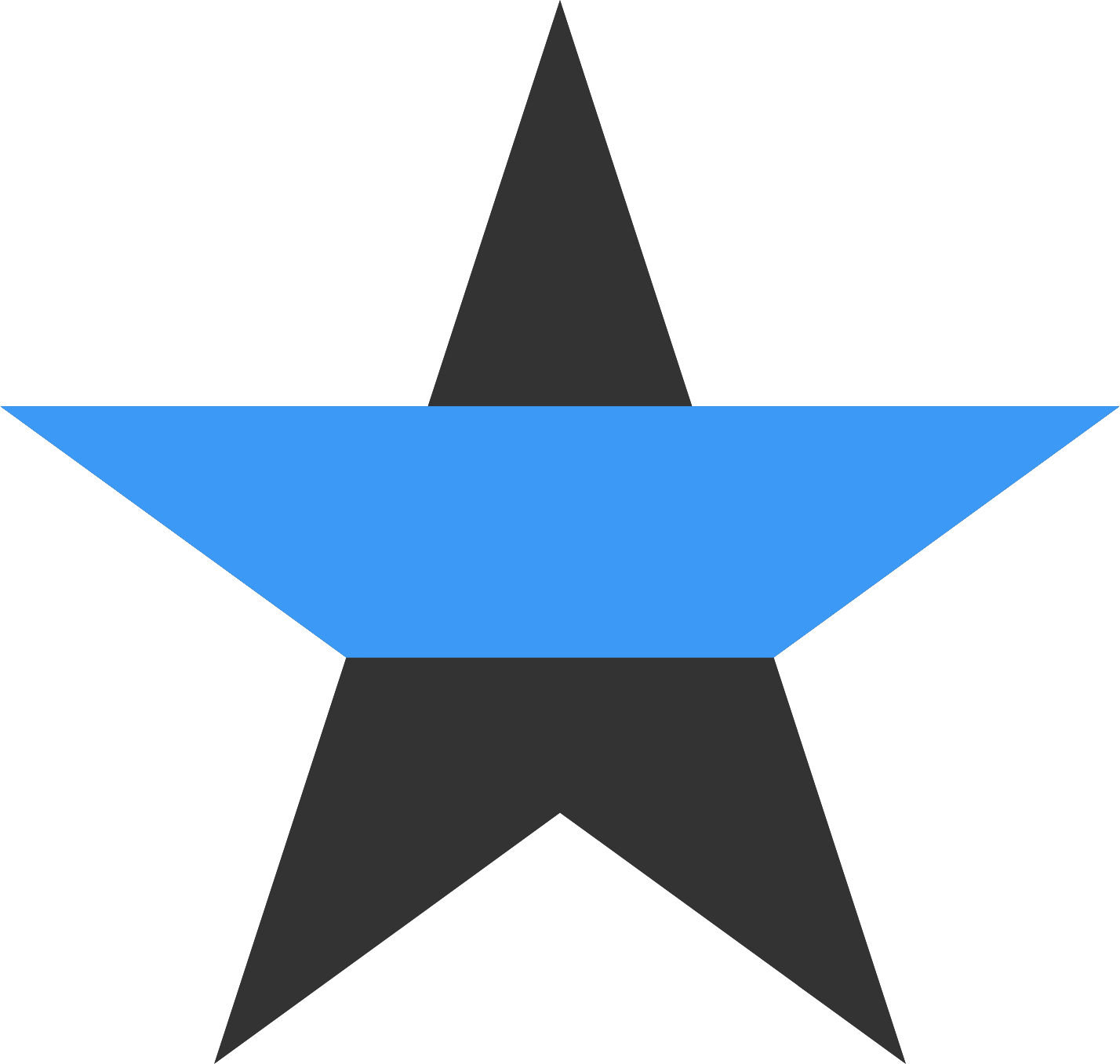In the regular star polygon above, $\text{\_\_\_\_\_\_\_\_\_\_\_}.$

×